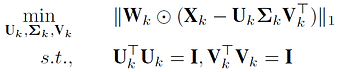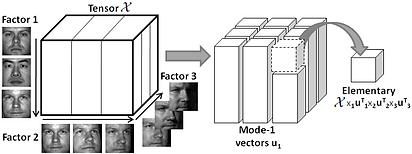###### Multifactor analysis for face recognition

S. Park and M. Savvides, “An extension of multifactor analysis for face recognition based on submanifold learning,” in Processings of IEEE Conference on Computer Vision and Pattern Recognition (CVPR), 2010, pp. 2645–2652.

S. Park and M. Savvides, “Multifactor analysis based on factor-dependent geometry,” in Processings of IEEE Conference on Computer Vision and Pattern Recognition (CVPR), 2011, pp. 2817–2824.

K. Luu, M. Savvides, T. D. Bui, and C. Y. Suen, “Compressed submanifold multifactor analysis with adaptive factor structures,” in Proceedings of IEEE International Conference on Pattern Recognition (ICPR), 2012, pp. 2715–2718.

K. Luu, M. Savvides, T.D.Bui and C.Y.Suen, "Compressed Submanifold Multifactor Analysis," IEEE Trans. on Pattern Analysis and Machine Intelligence (TPAMI), 2013. (under review)

Although widely used, Multilinear PCA (MPCA), one of the leading multilinear analysis methods, still suffers from four major drawbacks. First, it is very sensitive to outliers and noise. Second, it is unable to cope with missing values. Third, it is computationally expensive since MPCA deals with large multi-dimensional datasets. Finally, it is unable to maintain the local geometrical structures due to the averaging process. We proposes several advanced multifactor analysis approaches to solve the problems mentioned above. One of our approach, Compressed Submanifold Multifactor Analysis (CSMA), can deal with the problem of missing values and outliers in real-world data. The Random Projection method is used to obtain the fast low-rank approximation of a given multifactor dataset. In addition, it is able to preserve the geometry of the original data. Our CSMA method can be used efficiently for multiple purposes, e.g. noise and outlier removal, estimation of missing values, biometric applications. We show that CSMA method can achieve good results and is very efficient in the inpainting problem. Our method also achieves higher face recognition rates compared to Low Rank Tensor Completion (LRTC,) Submanifold Preserving Multifactor Analysis (SPMA), MPCA and some other subspace methods, i.e. Principal Component Analysis (PCA), Linear Discriminant Analysis (LDA) and Locality Preserving Projections (LPP), on three challenging face databases, i.e. CMU-MPIE, CMU-PIE and Extended YALE-B.

Higher Order SVD (HOSVD)

Higher order Singular Value Decomposition (SVD) is a multilinear generalization of SVD. Given an n order tensor X, HOSVD can decompose it into a core tensor Z and n orhthogonal matrices, i.e. a matrix U (for pixels) and n matrices Vk to present n factors. Without loss of generality, assume that n = 3, a tensor X can be decomposed using HOSVD as follows,

where k is the k-mode matrix product of a tensor. In MPCA, HOSVD is reformulated in terms of matrices instead of tensors, using the Kronecker product. The equivalent form in MPCA can be presented as follows,

where the matrix Z results from the pixel-mode flattening of a core tensor. Vk is the right singular vector matrix of the flattened tensor X along the factor k.

Noisy Data and Outliers Distortion
SVD is infeasible when the input data contains missing values. It is also sensitive to outliers. The problem of subspace estimation becomes equivalent to the optimization of the L2-norm cost function

where the first r columns of A presenting a subspace to minimize the defined L2-norm cost function, A = U(:; 1 : r) and B = V(:; 1 : r). SVD produces a closed form solution to the L2-norm cost function.

Factor Structures Deformation
One of PCA properties is that the low-dimensional subspaces obtained by PCA preserve the dot products between pairs of samples. The Gram matrices of X and Y_PCA are identical.

In order to further understand the properties of MPCA’s parameters, another equivalent approach is shown to compute the parameters from MPCA. In this approach, MPCA computation is based on averaging parts of the Gram matrix. A similar approach can be employed to find all Gram matrices Gi. The Gram matrix represents the Euclidean distances of all sample pairs. Therefore, factor-i parameters computed by Gi show the mean structure of the ni factor-i-variantsubmanifolds. MPCA aims to independently decompose the relationships of multifactors through the averaging process.

Therefore, in order to avoid the averaging process when computing Gram matrices Gi, Procrustes is then employed for local alignment to remove the differences in each coordinate while preserving structures of each manifold.

Multifactor L1-norm Decomposition

Instead of decomposing multi-factors using the regular L2-norm SVD which is unable to deal with missing data, SVD-L1 algorithm is proposed to decompose the relationships among these factors. In addition, the L1-based decomposition approach can avoid the outliers distortion.

Given a matrix Xk flattened from a tensor X along the factor k, the decomposition equation is reformulated by minimizing the following error,

where Uk, k and Vk are defined similarly to the ones in defined above. Far apart from SVD-L2, SVD-L1 allows to decompose X with missing values and outliers denoted by the weight matrix Wk flattened along the factor k from the weight tensor W, Wk(i; j) > 0 if the data point exists, otherwise Wk(i; j) = 0.